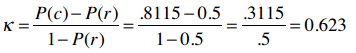# 第七章：朴素贝叶斯和文本数据

## 非结构化文本的分类算法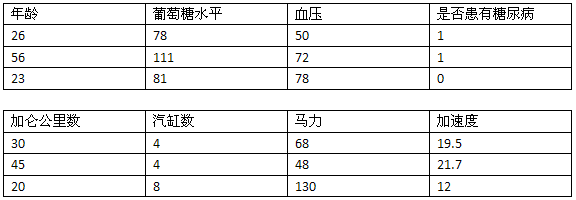### 自动判别文本中的感情色彩• 表达喜欢的词：美味、好吃、不错、喜欢、可口
• 表达厌恶的词：糟糕、难吃、不好、讨厌、恶心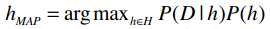argmax表示选取概率最大的分类；h∈H表示计算每个事件的概率；P(D|h)表示在给定h的条件下，D发生的概率（如给定某类文章，这类文章中特定单词出现的概率）；P(h)则指事件h发生的概率。

``````P(喜欢) = 0.5
P(讨厌) = 0.5
``````## 训练阶段

1. 将该分类下的所有文章合并到一起；
2. 统计每个单词出现的数量，记为n；
3. 对于总词汇表中的单词wk，统计他们在本类文章中出现的次数nk
4. 最后应用下方的公式：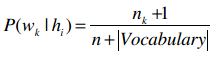## 使用朴素贝叶斯进行分类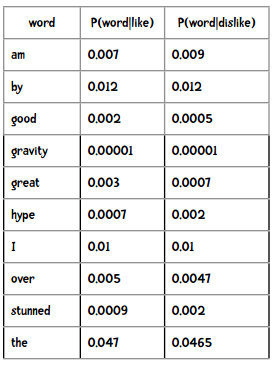I am stunned by the hype over gravity.

``````P(like)×P(I|like)×P(am|like)×P(stunned|like)×...
P(dislike)×P(I|dislike)×P(am|dislike)×P(stunned|dislike)×...
````````````>>> 0.0001 ** 100
0.0
``````

``````>>> import math
>>> p = 0
>>> for i in range(100):
...     p += math.log(0.0001)
...
>>> p
-921.034037197617
``````

• bn = x 可以转换为 logbx = n
• log10(ab) = log10(a) + log10(b)

## 新闻组语料库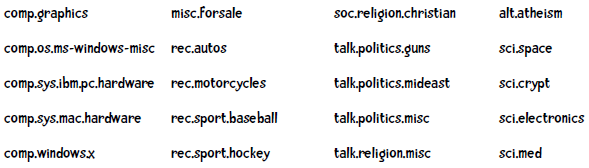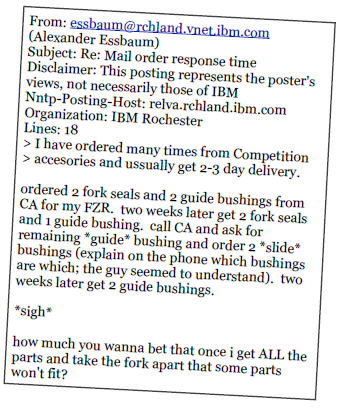### 把不要的东西丢掉！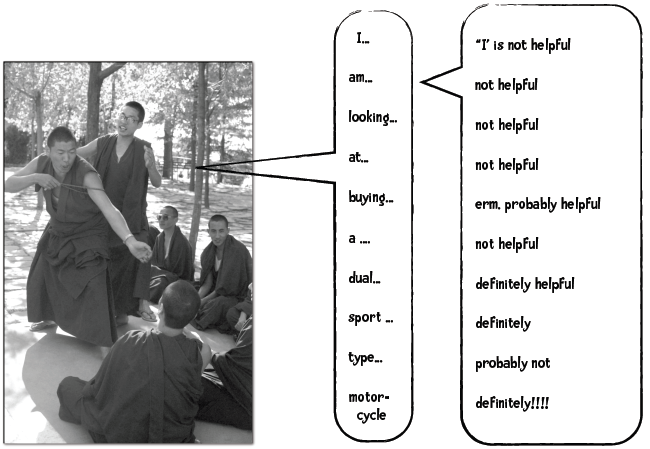1. 能够减少需要处理的数据量；
2. 这些词的存在会对分类效果产生负面影响。

### 常用词和停词### 编写Python代码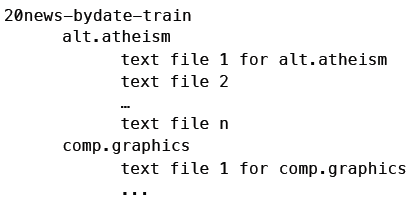1. 读取停词列表；
2. 获取训练集中各目录（分类）的名称；
3. 对于各个分类，调用train方法，统计单词出现的次数；
4. 计算下面的公式：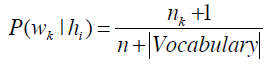``````from __future__ import print_function
import os, codecs, math

class BayesText:

def __init__(self, trainingdir, stopwordlist):
"""朴素贝叶斯分类器
trainingdir 训练集目录，子目录是分类，子目录中包含若干文本
stopwordlist 停词列表（一行一个）
"""
self.vocabulary = {}
self.prob = {}
self.totals = {}
self.stopwords = {}
f = open(stopwordlist)
for line in f:
self.stopwords[line.strip()] = 1
f.close()
categories = os.listdir(trainingdir)
# 将不是目录的元素过滤掉
self.categories = [filename for filename in categories
if os.path.isdir(trainingdir + filename)]
print("Counting ...")
for category in self.categories:
print('    ' + category)
(self.prob[category],
self.totals[category]) = self.train(trainingdir, category)
# 删除出现次数小于3次的单词
toDelete = []
for word in self.vocabulary:
if self.vocabulary[word] < 3:
# 遍历列表时不能删除元素，因此做一个标记
toDelete.append(word)
# 删除
for word in toDelete:
del self.vocabulary[word]
# 计算概率
vocabLength = len(self.vocabulary)
print("Computing probabilities:")
for category in self.categories:
print('    ' + category)
denominator = self.totals[category] + vocabLength
for word in self.vocabulary:
if word in self.prob[category]:
count = self.prob[category][word]
else:
count = 1
self.prob[category][word] = (float(count + 1)
/ denominator)
print ("DONE TRAINING\n\n")

def train(self, trainingdir, category):
"""计算分类下各单词出现的次数"""
currentdir = trainingdir + category
files = os.listdir(currentdir)
counts = {}
total = 0
for file in files:
#print(currentdir + '/' + file)
f = codecs.open(currentdir + '/' + file, 'r', 'iso8859-1')
for line in f:
tokens = line.split()
for token in tokens:
# 删除标点符号，并将单词转换为小写
token = token.strip('\'".,?:-')
token = token.lower()
if token != '' and not token in self.stopwords:
self.vocabulary.setdefault(token, 0)
self.vocabulary[token] += 1
counts.setdefault(token, 0)
counts[token] += 1
total += 1
f.close()
return(counts, total)
``````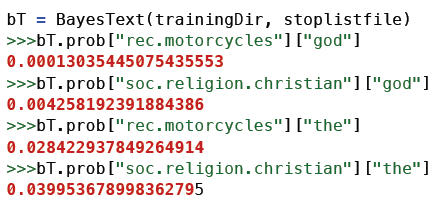god这个词在rec.motorcycles新闻组中出现的概率是0.00013，而在soc.religion.christian新闻组中出现的概率是0.00424。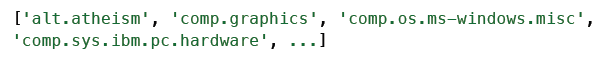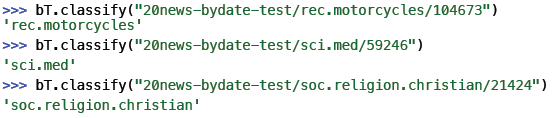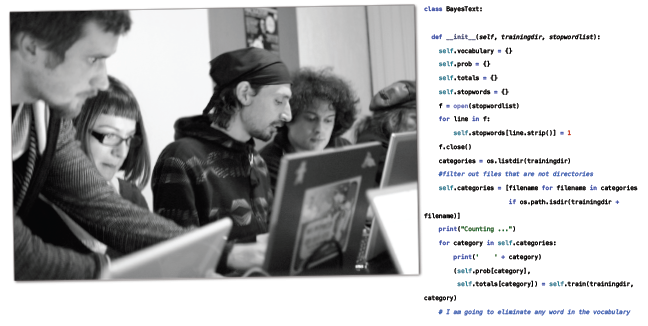``````    def classify(self, filename):
results = {}
for category in self.categories:
results[category] = 0
f = codecs.open(filename, 'r', 'iso8859-1')
for line in f:
tokens = line.split()
for token in tokens:
#print(token)
token = token.strip('\'".,?:-').lower()
if token in self.vocabulary:
for category in self.categories:
if self.prob[category][token] == 0:
print("%s %s" % (category, token))
results[category] += math.log(
self.prob[category][token])
f.close()
results = list(results.items())
results.sort(key=lambda tuple: tuple, reverse = True)
# 如果要调试，可以打印出整个列表。
return results
``````

``````    def testCategory(self, directory, category):
files = os.listdir(directory)
total = 0
correct = 0
for file in files:
total += 1
result = self.classify(directory + file)
if result == category:
correct += 1
return (correct, total)

def test(self, testdir):
"""测试集的目录结构和训练集相同"""
categories = os.listdir(testdir)
# 过滤掉不是目录的元素
categories = [filename for filename in categories if
os.path.isdir(testdir + filename)]
correct = 0
total = 0
for category in categories:
print(".", end="")
(catCorrect, catTotal) = self.testCategory(
testdir + category + '/', category)
correct += catCorrect
total += catTotal
print("\n\nAccuracy is  %f%%  (%i test instances)" %
((float(correct) / total) * 100, total))
``````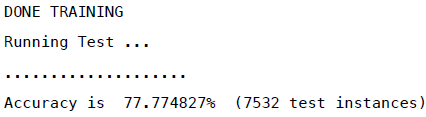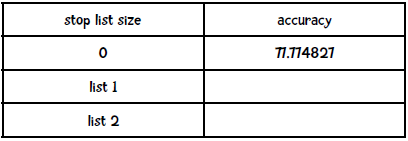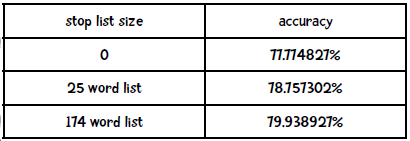## 朴素贝叶斯与情感分析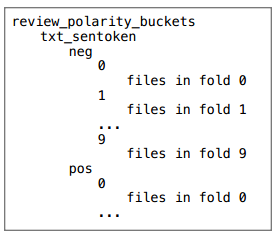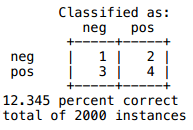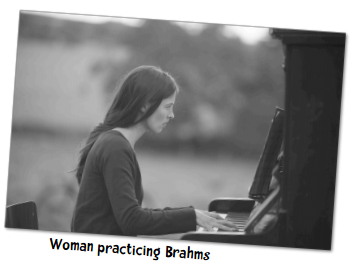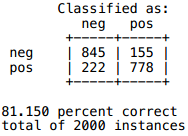Kappa指标则是：